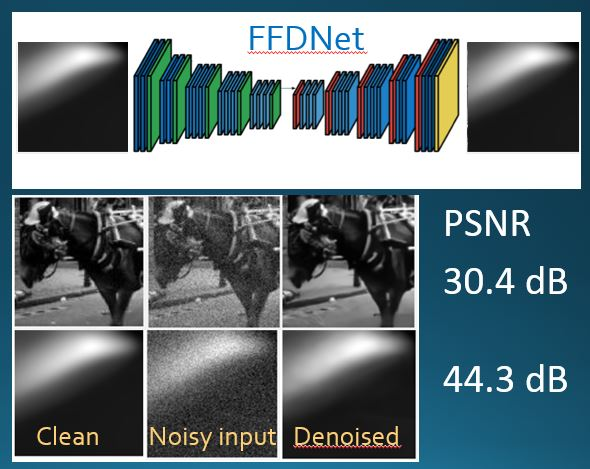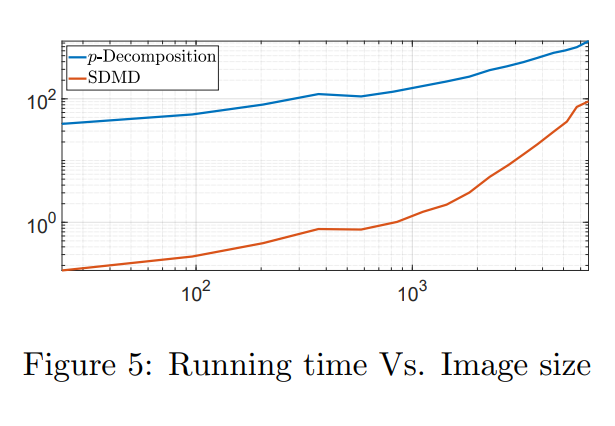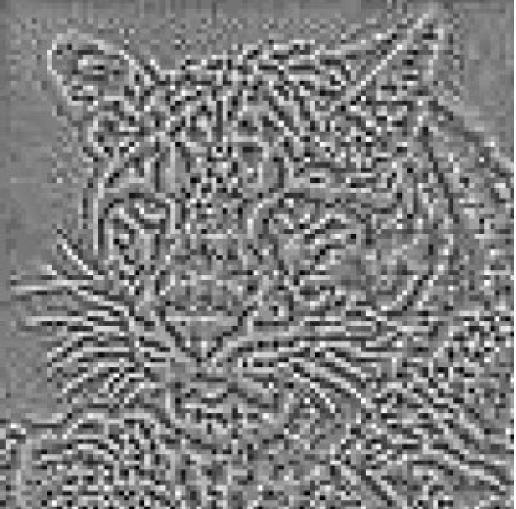# Total-Variation Mode Decomposition

Ido Cohen, Tom Berkov, Guy Gilboa, “Total-Variation Mode Decomposition”,  Proc. SSVM 2021, pp. 52-64

Abstract

In this work we analyze the Total Variation (TV) flow applied to one dimensional signals. We formulate a relation between Dynamic Mode Decomposition (DMD), a dimensionality reduction method based on the Koopman operator, and the spectral TV decomposition. DMD is adapted by time rescaling to fit linearly decaying processes, such as the TV flow. For the flow with finite subgradient transitions, a closed form solution of the rescaled DMD is formulated. In addition, a solution to the TV-flow is presented, which relies only on the initial condition and its corresponding subgradient. A very fast numerical algorithm is obtained which solves the entire flow by elementary subgradient updates.

Bibtex Citation

@InProceedings{10.1007/978-3-030-75549-2_5,
author="Cohen, Ido
and Berkov, Tom
and Gilboa, Guy",
editor="Elmoataz, Abderrahim
and Fadili, Jalal
and Qu{\'e}au, Yvain
and Rabin, Julien
and Simon, Lo{\"i}c",
title="Total-Variation Mode Decomposition",
booktitle="Scale Space and Variational Methods in Computer Vision",
year="2021",
publisher="Springer International Publishing",
address="Cham",
pages="52--64",
}# Nonlinear Spectral Processing of Shapes via Zero-homogeneous Flows

Jonathan Brokman, Guy Gilboa, Proc. SSVM, “Nonlinear Spectral Processing of Shapes via Zero-homogeneous Flows”, pp. 40-51, 2021

Abstract

In this work we extend the spectral total-variation framework, and use it to analyze and process 2D manifolds embedded in 3D. Analysis is performed in the embedding space – thus “spectral arithmetics” manipulate the shape directly. This makes our approach highly versatile and accurate for feature control. We propose three such methods, based on non-Euclidean zero-homogeneous p-Laplace operators. Each method satisfies distinct characteristics, demonstrated through smoothing, enhancing and exaggerating filters.

Cite

Brokman J., Gilboa G. (2021) Nonlinear Spectral Processing of Shapes via Zero-Homogeneous Flows. In: Elmoataz A., Fadili J., Quéau Y., Rabin J., Simon L. (eds) Scale Space and Variational Methods in Computer Vision. SSVM 2021. Lecture Notes in Computer Science, vol 12679. Springer, Cham. https://doi.org/10.1007/978-3-030-75549-2_4# Nonlinear Power Method for Computing Eigenvectors of Proximal Operators and Neural Networks

Accepted to SIAM J. on Imaging Scienes, 2021

Leon Bungert,  Ester Hait-Fraenkel , Nicolas Papadakis and Guy Gilboa, arXiv

Neural networks have revolutionized the field of data science, yielding remarkable solutions in a data-driven manner.  For instance, in the field of mathematical imaging, they have surpassed traditional methods based on convex regularization. However, a fundamental theory supporting the practical applications is still in the early stages of development. We take a fresh look at neural networks and examine them via nonlinear eigenvalue analysis. The field of nonlinear spectral theory is still emerging, providing insights about nonlinear operators and systems.  In this paper we view a neural network as a complex nonlinear operator and attempt to find its nonlinear eigenvectors.  We first discuss the existence of such eigenvectors and analyze the kernel of $\relu$ networks. Then we study a nonlinear power method for generic nonlinear operators. For proximal operators associated to absolutely one-homogeneous convex regularization functionals, we can prove convergence of the method to an eigenvector of the proximal operator. This motivates us to apply a nonlinear method to networks which are trained to act similarly as a proximal operator. In order to take the non-homogeneity of neural networks into account we define a modified version of the power method.

We perform extensive experiments on various shallow and deep neural networks designed for image denoising. For simple nets, we observe the influence of training data on the eigenvectors.  For state-of-the-art denoising networks, we show that eigenvectors can be interpreted as (un)stable modes of the network, when contaminated with noise or other degradations.# Modes of Homogeneous Gradient Flows

Accepted to SIAM J. on Imaging Sciences, 2021

Ido Cohen, Omri Azencot, Pavel Lifshitz, Guy Gilboa, arXiv, July 2020

Finding latent structures in data is drawing increasing attention in broad and diverse fields such as fluid dynamics, signal processing, and machine learning. In this work, we formulate Dynamic Mode Decomposition (DMD) for two types of dynamical system. The first, a system which is derived by a $\gamma$-homogeneous operator ($\gamma\neq 1$).
The second, a system which can be represented as a symmetric operator.

Regarding to the first type, dynamical systems, derived by $\gamma$-homogeneous operators $\gamma\in[0,1)$, reach the steady state in finite time. This inherently contradicts the DMD model, which can be seen as an exponential data fitting algorithm. Therefore, the induced DMD operator leads to artifacts in the decomposition. We show certain cases where the DMD does not even exist. For homogeneous systems ($\gamma\neq 1$), we suggest a time rescaling that solves this conflict and show that DMD can perfectly restore the dynamics even for nonlinear flows. For dynamics which derived by a symmetric operator, we expect the eigenvalues of the DMD to be real. This requirement is embeded in a variant of the DMD algorithm, termed as Symmetric DMD  (SDMD).

With these adaptations, we formulate a closed form solution of DMD for dynamics $u_t = P(u)$, $u(t=0)=u_0$, where $P$ is a nonlinear $\gamma$-homogeneous operator, when the initial condition $u_0$ admits the nonlinear eigenvalue problem $P(u_0)=\lambda u_0$ ($u_0$ is a nonlinear eigenfunction, with respect to the operator $P$). We show experimentally that, for such systems, for any initial condition, SDMD achieves lower mean square error for the spectrum estimation. Finally, we formulate a discrete decomposition, related to nonlinear eigenfunctions of $\gamma$-homogeneous operator.# Revealing stable and unstable modes of denoisers through nonlinear eigenvalue analysis

### Ester Hait-Fraenkel, Guy Gilboa, arXiv

In this paper, we propose to analyze stable and unstable modes of generic image denoisers through nonlinear eigenvalue analysis. We attempt to find input images for which the output of a black-box denoiser is proportional to the input. We treat this as a nonlinear eigenvalue problem. This has potentially wide implications, since most image processing algorithms can be viewed as generic nonlinear operators. We introduce a generalized nonlinear power-method to solve eigenproblems for such black-box operators. Using this method we reveal stable modes of nonlinear denoisers. These modes are optimal inputs for the denoiser, achieving superior PSNR in noise removal. Analogously to the linear case (low-pass-filter), such stable modes are eigenfunctions corresponding to large eigenvalues, characterized by large piece-wise-smooth structures. We also provide a method to generate the complementary, most unstable modes, which the denoiser suppresses strongly. These modes are textures with small eigenvalues. We validate the method using total-variation (TV) and demonstrate it on the EPLL denoiser (Zoran-Weiss). Finally, we suggest an encryption-decryption application.# Experts with Lower-Bounded Loss Feedback: A Unifying Framework

Eyal Gofer, Guy Gilboa,  arxiv preprint

The most prominent feedback models for the best expert problem are the full information and bandit models. In this work we consider a simple feedback model that generalizes both, where on every round, in addition to a bandit feedback, the adversary provides a lower bound on the loss of each expert. Such lower bounds may be obtained in various scenarios, for instance, in stock trading or in assessing errors of certain measurement devices. For this model we prove optimal regret bounds (up to logarithmic factors) for modified versions of Exp3, generalizing algorithms and bounds both for the bandit and the full-information settings. Our second-order unified regret analysis simulates a two-step loss update and highlights three Hessian or Hessian-like expressions, which map to the full-information regret, bandit regret, and a hybrid of both. Our results intersect with those for bandits with graph-structured feedback, in that both settings can accommodate feedback from an arbitrary subset of experts on each round. However, our model also accommodates partial feedback at the single-expert level, by allowing non-trivial lower bounds on each loss.# Iterative Methods for Computing Eigenvectors of Nonlinear Operators

Guy Gilboa, arXiv preprint

A chapter to appear in Handbook of Mathematical Models and Algorithms in Computer Vision and Imaging.

In this chapter we are examining several iterative methods for solving nonlinear eigenvalue problems. These arise in variational image-processing, graph partition and classification, nonlinear physics and more. The canonical eigenproblem we solve is $T(u)=\lambda u$, where $T:R^n\to R^n$ is some bounded nonlinear operator. Other variations of eigenvalue problems are also discussed. We present a progression of 5 algorithms, coauthored in recent years by the author and colleagues. Each algorithm attempts to solve a unique problem or to improve the theoretical foundations. The algorithms can be understood as nonlinear PDE’s which converge to an eigenfunction in the continuous time domain. This allows a unique view and understanding of the discrete iterative process. Finally, it is shown how to evaluate numerically the results, along with some examples and insights related to priors of nonlinear denoisers, both classical algorithms and ones based on deep networks.# NeurIPS 2020: Deeply Learned Spectral Total Variation Decomposition

Tamara G. Grossmann, Yury Korolev, Guy Gilboa, Carola-Bibiane Schönlieb, arXiv 2020

Accepted for NeurIPS 2020.

Non-linear spectral decompositions of images based on one-homogeneous functionals such as total variation have gained considerable attention in the last few years. Due to their ability to extract spectral components corresponding to objects of different size and contrast, such decompositions enable filtering, feature transfer, image fusion and other applications. However, obtaining this decomposition involves solving multiple non-smooth optimisation problems and is therefore computationally highly intensive. In this paper, we present a neural network approximation of a non-linear spectral decomposition. We report up to four orders of magnitude (×10,000) speedup in processing of mega-pixel size images, compared to classical GPU implementations. Our proposed network, TVSpecNET, is able to implicitly learn the underlying PDE and, despite being entirely data driven, inherits invariances of the model based transform. To the best of our knowledge, this is the first approach towards learning a non-linear spectral decomposition of images. Not only do we gain a staggering computational advantage, but this approach can also be seen as a step towards studying neural networks that can decompose an image into spectral components defined by a user rather than a handcrafted functional.# Adaptive LiDAR Sampling and Depth Completion using Ensemble Variance

Eyal Gofer, Shachar Praisler, Guy Gilboa,  arXiv July, 2020

Project details

Abstract

This work considers the problem of depth completion, with or without image data, where an algorithm may measure the depth of a prescribed limited number of pixels. The algorithmic challenge is to choose pixel positions strategically and dynamically to maximally reduce overall depth estimation error. This setting is realized in daytime or nighttime depth completion for autonomous vehicles with a programmable LiDAR. Our method uses an ensemble of predictors to define a sampling probability over pixels. This probability is proportional to the variance of the predictions of ensemble members, thus highlighting pixels that are difficult to predict. By additionally proceeding in several prediction phases, we effectively reduce redundant sampling of similar pixels. Our ensemble-based method may be implemented using any depth-completion learning algorithm, such as a state-of-the-art neural network, treated as a black box. In particular, we also present a simple and effective Random Forest-based algorithm, and similarly use its internal ensemble in our design. We conduct experiments on the KITTI dataset, using the neural network algorithm of Ma et al. and our Random Forest based learner for implementing our method. The accuracy of both implementations exceeds the state of the art. Compared with a random or grid sampling pattern, our method allows a reduction by a factor of 4-10 in the number of measurements required to attain the same accuracy.# Presentations at SIAM Conference on Imaging Science

SIAM Conf on Imaging Sciences 2020 was held virtually this year.

Ety and Ido presented talks at the minisymposium Nonlinear Spectral Analysis with Applications in Imaging and Data Science (a minisymposium organized by Leon Bungert, Ido Cohen and Guy Gilboa)

Jonathan and Guy presented a joint work at the minisymposium Are We Ready for Semi-Supervised Learning for Big Data? From Theory to Practice